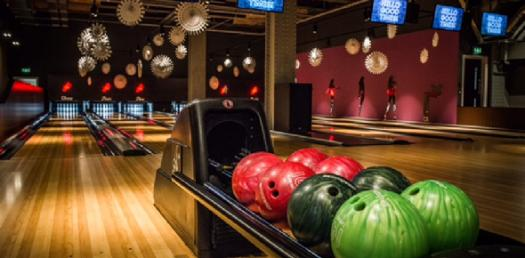23 Questions | Total Attempts: 102Settings4th grade science study test for Bowling's science classes

• 1.
A condition that is NOT changed in a scientific investigation is called a/an  __________________
• A.

Variable

• B.

Constant

• C.

Hypothesis

• D.

Experiment

• 2.
A scientific investigation in which one variable is changed and all the others are kept the same is a/an ______________________
• A.

Controlled experiment

• B.

Scientific method

• C.

Hypothesis

• D.

Constant

• 3.
A set of steps designed to test a specific hypothesis is a/an
• A.

Scientific method

• B.

Variable

• C.

Standard

• D.

Experiment

• 4.
A statement of what you think will happen in an experiment and why is called a/an ______________________
• A.

Observation

• B.

Constant

• C.

Scientific method

• D.

Hypothesis

• 5.
An untested conclusion based on observations in a/an _______________________
• A.

Inference

• B.

Hypothesis

• C.

Variable

• D.

Scientific method

• 6.
A tool with several lenses that can magnify an object many times is a/an _________________.
• A.

Magnifying lens

• B.

Bug box

• C.

Microscope

• D.

Ruler

• 7.
The set of steps scientists follow to figure out how things work and affect each other is the ______________________
• A.

Hypothesis

• B.

Scientific method

• C.

Inference

• D.

Controlled experiment

• 8.
An accepted measure is a/an __________________
• A.

Standard measure

• B.

Variable measure

• C.

Constant measure

• D.

Inferred measure

• 9.
An element in an experiment that can be changed is a/an _________________
• A.

Experiment

• B.

Constant

• C.

Hypothesis

• D.

Variable

• 10.
The amount of space a liquid takes up is its ___________________
• A.

Height

• B.

Volume

• C.

Width

• D.

Length

• 11.
A ruler, a flexible  tape measure and a meter stick are all tools for __________________
• A.

Measurings volume

• B.

Measuring length

• C.

Measuring weight

• D.

Measuring temperature

• 12.
Graduated cylinders, beakers, and droppers are all tools for _________________ of liquids
• A.

Measuring volume

• B.

Measuring length

• C.

Measuring weight

• D.

Measuring temperature

• 13.
A thermometer is a tool used for _____________________________
• A.

Measuring volume

• B.

Measuring length

• C.

Measuring weight

• D.

Measuring temperature

• 14.
Mass can be measured by using a ________________________
• A.

• B.

Thermometer

• C.

Ruler

• D.

Pan balance

• 15.
The pull of gravity on an object can be measured by a _________________________.
• A.

Spring scale

• B.

Meter stick

• C.

Dropper

• D.

Microscope

• 16.
The tool for observing that magnifies objects several times is a/an _________________________
• A.

Magnifying lens

• B.

Bug box

• C.

Microscope

• D.

Glass

• 17.
The tool for observing that makes objects appear larger is a/an ______________________________.
• A.

Glass

• B.

Microscope

• C.

Bug box

• D.

Magnifying lens

• 18.
A tool used for observing live insects is a _____________________.
• A.

Microscope

• B.

Magnifying lens

• C.

Bug box

• D.

Glass

• 19.
Tweezers and forceps are tools used for __________________.
• A.

Measuring

• B.

Handling

• C.

Observing

• D.

Classifying

• 20.
When you use your senses to gather information, you are _____________________.
• A.

Observing

• B.

Inferring

• C.

Predicting

• D.

Comparing

• 21.
When you observe and form an untested conclusion based on your observations, you are _____________________.
• A.

Observing

• B.

Inferring

• C.

Predicting

• D.

Comparing

• 22.
When you describe the ways things are alike and ways they are different, you are ___________________.
• A.

Observing

• B.

Inferring

• C.

Predicting

• D.

Comparing

• 23.
When you use your knowledge to guess what will happen next, you are ________________________.
• A.

Observing

• B.

Inferring

• C.

Predicting

• D.

Comparing

Related TopicsBack to top